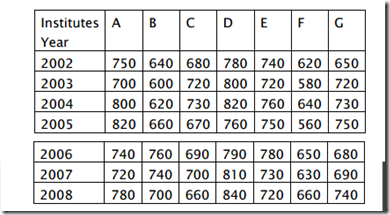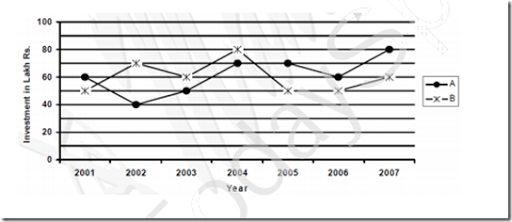Saturday, 13 May 2017

1 .

Directions (1 - 5):Study the following graph carefully to answer these questions.
Strength (number of students) of seven institute over the years$Q.$ What is the ratio between total strength of institute A, B, and C together in year 2003 and the total strength of institute E, F and G together in 2005 respectively?

 A.   103:101 B.   101:103 C.   51:53 D.   53:51
2 . If from institute B, overall 60% students passed for all the given years, approximately what is the average number of students passed?

 A.   430 B.   425 C.   390 D.   405
3 . If the year 2002, the overall percentage of students passed from all the institute is 70% total how many students passed in 2002 from all the institutes together?

 A.   3402 B.   3420 C.   3422 D.   3382
4 . Strength of institute F in the year 2004 is what percentage of the total strength of that institute for all seven years together? (Round off to two digits after decimal)

 A.   14.28 B.   14.98 C.   12.90 D.   14.75
5 . What is the difference between the total number of students in 2006 for all the institute together and total number of students in the year 2008 for all the institute together?

 A.   50 B.   70 C.   10 D.   30
6 . Q. (6 - 10): Study the following graph carefully to answer these questions.
INVESTMENTS (IN LAKH RS.) OF TWO BUSINESS PARTNERS A & B OVER THE YEARS$Q.$ What was the percent rise in A’s investment in the year 2004 from the previous year?

1.25%
2.20%
3.33$1\over3$%
4.None of these

 A.   1 B.   2 C.   3 D.   4
7 . What was the percent in investment of B in the year 2004 from 2001?

 A.   45.6 B.   37.5 C.   30 D.   60
8 . What was the percent rise/fall in the total investment of A & B together from the year 2002 to 2005? (Rounded off to two digits after decimal)

 A.   8.33% fall B.   9.09% rise C.   8.33% rise D.   9.09% fall
9 . What is the ratio between total investment of A in the year 2001, 2002 and 2003 together and the total investment of B in these years together respectively?

 A.   5:6 B.   6:5 C.   15:17 D.   17:15
10 . Investment of B in the year 2003 is approximately what percent of his total investment for all the years together?

 A.   12 B.   18 C.   20 D.   14
1 .
 Answer : Option B Explanation : Total strength of institute A, B & C in 2003= 700 + 600 + 720= 2020Total strength of institutes E, F & G in 2005= 750 + 560 + 750 = 2060∴ ratio =2020 : 2060= 202:206= 101:103
2 .
 Answer : Option D Explanation : Total students in institute B= 640 + 600 + 620 + 660 + 760 + 740 + 700= 472060% of 4720 = 2832Average = $2832 \over 7$= 404.77 or 405.
3 .
 Answer : Option A Explanation : Strength of students passed in 2002 from all the institute together= 750 + 640 + 680 + 780 + 740 + 620 + 650= 486070% of 4860 = 3402.
4 .
 Answer : Option D Explanation : Strength of Institute F, in 2004 = 640Total strength in institute F= 620 + 580 + 640 + 560 + 650 + 630 + 660= 4340$640\over4340$ x 100= 14.75%
5 .
 Answer : Option C Explanation : Total students in 2006 from all the institute together = 740 + 760 + 690 + 780 + 650 + 680= 5090Total students in 2008 from all the institute together.= 780 + 700 + 660 + 840 + 720 + 660 + 740= 5100∴ Difference = 5100 – 5090= 10
6 .
 Answer : Option D Explanation : % rise in A’s investment in 2004 from previous year= $50-70\times100\over50$ = 40%
7 .
 Answer : Option D Explanation : Explanation: - % rise investment of B in 2004 from 2001= $80-50\over50$ x 100 = $30\over 50$ x 100= 60%
8 .
 Answer : Option B Explanation : Total investment of A&B in 2002 = 40+70 = 110Total investment of A&B in 2005 = 50+70 = 120Therefore, $120-110\over110$ x 100 = $10\over110$ x 100 = 9.09% increase
9 .
 Answer : Option A Explanation : Total investment of A in 2001, 2002 & 2003= 60 + 40 + 50 = 150Total investment of B in 2001, 2002 & 2003= 50 + 70 + 60= 180∴ Ratio = 150 : 180 = 15:18= 5:6
10 .
 Answer : Option D Explanation : Investment of B in 2003 = 60Total investment of B for all the years together= 50 + 70 + 60 + 80 + 50 + 50 + 60= 420Therefore, $60\over 420$ x 100 = 14% (approx.)